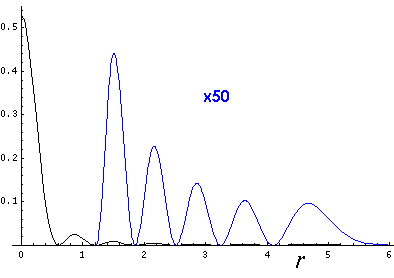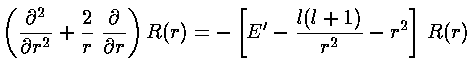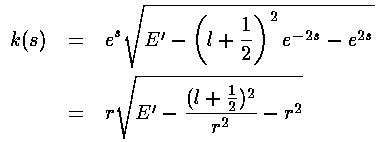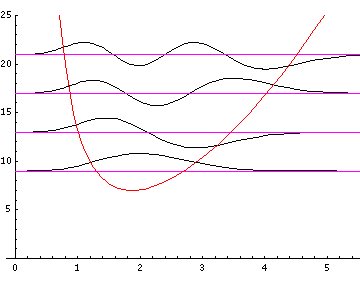## SHM p.14

### WKB approximation

You should have noticed that the wavefunctions presented in the 2-d and 3-d cases did not follow the 1-d wavefunctions rules (e.g., that where the classical velocity is slow, the wavefunction is large). For example, recall that in order to see the nr=6,l=0,m=0 wavefunction near its classical turning point we needed to magnify:Qualitatively speaking, the ever smaller wavefunction as you move away from the origin is the result of flux conservation: an outgoing wave's probability amplitude is spread out over ever larger surface as r increases.

Mathematically we can see this as a breakdown of the assumptions of the WKB approximation:

• WKB requires a differential equation of this form:whereas the R differential equation for the 3-d oscillator is:• WKB seeks a wavefunction that goes to zero at ±infinity, whereas (generally) R(r=0)=0.
We can quickly solve the first problem:Thus the new "wavefunction" u=rsatisfies the WKB-like differential equation.

In order to solve the second problem we must perform a change of variables to move the zero of u at r=0 out to minus infinity. The transformation s=ln(r) does just what we want.However, the differential equation for u in terms of s is no longer in the WKB form:Thus is solving second problem, we've screwed up the first and must now work to again get the differential equation into WKB form:Putting these results into our differential equation finally gives us a WKB form in s (and hence with WKB behavior at infinity).We can now read off the position dependent wavenumber kNote that essentially what has happened is the centrifugal barrier term l(l+1)/r2 has become (l+½)2/r2, a small correction of order l-2.

The wavefunction v is given by:The integral of k can be rearranged to give:Now to get the WKB estimates for the eigenenergies we must integrate k through the allowed region. The allowed region should include n+½ half-waves, i.e., a phase of(n+½). With a little work on the complex plane we can evaluate that integral exactly:exactly reproducing the correct result:

E'=4nr+2l+3

So the bottom line is that if you plot ralong with the effective potential with (l+½)2 rather than l(l+1) things should look as in the 1-d case. In fact, this is how you will find most books displaying 3-d wavefunctions. Here is a repeat stacked wavefunction plot (for l=0,1,3) following this formula.Here is the nr=32 l=0 (probability density)×r2 plotted along with the WKB result (in red):Note that while these plots look more "normal" they hide the fact that the probability density is highest near the origin, rather than at the far r turning point.